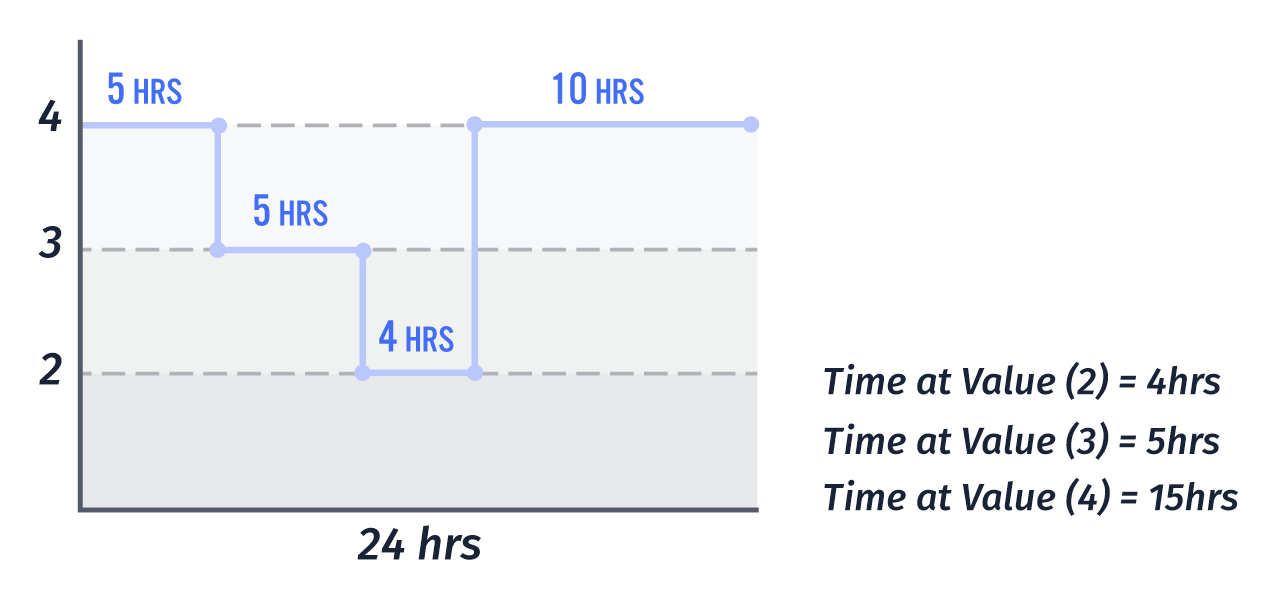# Data Aggregations

When utilizing state data for Workflows, Dashboards, and Notebooks, there are a number of aggregation methods that can be applied to raw data. However, not all aggregation methods are available for all attribute data types.

The following data set will be used to describe the aggregation methods listed below: `[41, 80, 7, 90, 40, 89, 33, 21, 63]`

## All Data Types

These methods can be applied to all data types:

### Count

Returns the total number of data points within the aggregation bucket.

In our example, `Count` will return `9`, which is the total number of data points in the dataset.

### First

Returns the first received (oldest) data point within the aggregation bucket.

For our dataset, `First` will return `41`, which is the first number in the dataset.

### Last

Returns the last received (most recent) data point within the aggregation bucket.

Here, `Last` will return `63`, or the last number in the dataset.

### Time At ValueReturns the total time (in milliseconds) that an attribute equals a value within a given range of time. This method is not valid for attribute calculation of System devices. For multiple devices, the total time is averaged.

For example, return the number of times an attribute reported any value within a given time range. In the diagram above, the time spent at value 3 was 5 hours.

For this aggregation, an additional argument must be specified.

Matching Value: Attribute value to match within a time range for calculating the time at that value.

## Number and Boolean Aggregations

The methods below are only available for number and boolean data types.

For booleans, `true` values are cast to the number `1` and `false` values are cast to `0` when calculating these results.

### Max

Returns the maximum (highest) value of all points within the aggregation bucket.

In the dataset above, the `Max` will be reported as `90`.

### Min

Returns the minimum (lowest) value of all points within the aggregation bucket.

Using the dataset, the `Min` value will be `7`.

### Median

Returns the median (middlemost) value of all points within the aggregation bucket.

In the example dataset, the `Median` will be `40`.

### Mean

Returns the mean, or average, of all values within the aggregation bucket.

The `mean` for the example dataset will be calculuated as `51.5556`.

### Sum

Returns the summation of all values within the aggregation bucket.

The `sum` of the example dataset will be calculated as `464`.

### Standard Deviation

Returns the sample standard deviation of the aggregation bucket.

The `Standard Deviation` will be calculated as `30.2659508`.Need Help? Your feedback and questions are very important to us. Let us know in the WEGnology Forums.